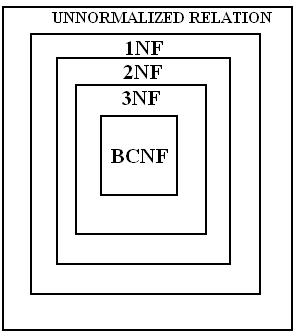# DATABASE & SQL/PLSQL

NORMALIZATION
 Previous Home Next

Some rules that should followed to achieve a good database design are:

1. Each table should have an identifier.
2. Each table should store data for a single type entity
3. Columns that should store data for a single type of entity.
4. The repetition of values or columns should be avoided.

To remove the redundancy of a table as called Normalization. In other words duplicity or repetitions of data never occur in database. Normalization is mainly minimizing redundancy, insertion, deletion and update anomalies. Normalization achieve through functional dependency. Normalization is much type.

### Functional Dependency:

Functional dependencies (FDs) are used to specify formal measures of the goodness of relational designs and used to define normal forms for relations. FDs are constraints that are derived from the meaning and interrelationships of the data attributes. FDs are derived from the real-world constraints on the attributes

### Example:

A set of attributes X functionally determines a set of attributes Y if the value of X determines a unique value for Y X--> Y holds if whenever two tuples have the same value for X, they must have the same value for Y If t1[X] =t2[X], then t1[Y] =t2[Y] in any relation instance r(R) X-->Y in R specifies a constraint on all relation instances r(R)

### Fully Functional dependency:

A functional dependency X --> Y is full functional dependency if any attribute A removed from X. It means that the dependency does not hold any more then it is not Fully Functional dependence. Means each attribute is functionally dependent.

All categories are in sequential order:

1. 1NF: There is no repetition of values and data in table known as 1NF. In other words the 1NF disallows composite attributes, multivalued attributes, and nested relations, attributes whose values for an individual tuple are non-atomic.
2. 2NF: A relation schema R is in 2NF when it is in 1NF and every non-prime attribute A in R is fully functionally dependent on primary key.
3. 3NF: A relation schema R is in 3NF ,It is in 2NF and no non-prime attribute A in R is transitively dependent on the primary key. Transitive dependent means if there a set of attribute Z. that are neither a primary or candidate key and both X-->Z and Y-->Z holds.
4. BCNF: 3NF inadequate in some situation then it was not satisfactory for the table:
1. That had multiple candidate keys.
2. Where the multiple candidate keys were composite.
3. Where the multiple candidate keys were overlapped.

A relation schema R is in BCNF, It is in 3NF and additional constraints that for every FD X -> A, X must be a candidate key. "A relation is in the Boyce-Codd normal form (BCNF) if and only if every determinant is a candidate key.

5. 4NF: A relation schema R is said to be in 4NF, it is in BCNF and for every Multivalued dependency X --> Y that holds over R, Either X is subset or equal to (or) XY = R. or X is a super
6. 5NF: A relation schema R is said to be in 5 NF, it is in 4NF and relation schema R is said to be 5NF if for every join dependency {R1, R2, ..., Rn} that holds R, one the following is true Ri = R for some i. and The join dependency is implied by the set of FD, over R in which the left side is key of R.### Demoralization

The intentional introduction of redundancy in a table in order to improve performance is called demoralization. The decision to demoralize results in a trade-off performance and data integrity. Demoralization increases disk utilization.

 Previous Home Next